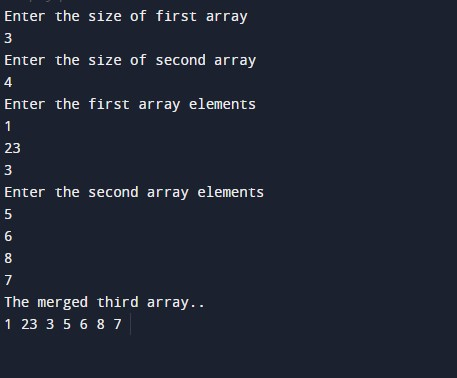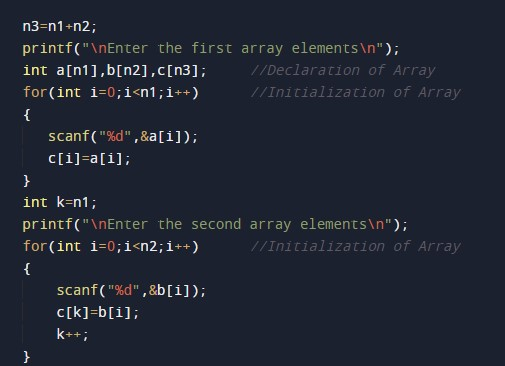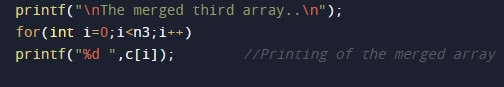# C Program For Merging Of Two Arrays

In this tutorial you will learn about the C Program For Merging Of Two Arrays and its application with practical example.

## C Program to Merge Two Arrays

In this tutorial, we will learn to create a C program that will merge two different array the element of Array using C programming.

## Prerequisites

Before starting with this tutorial we assume that you are best aware of the following C programming topics:

• Operators in C Programming.
• Basic Input and Output function in C Programming.
• Basic C programming.
• For loop in C Programming.

## Merging of two arrays:-

As we all know array is a collection of similar data type elements. In array only one variable is declared which can store multiple values. First will take the number of elements of array1 from the user. Then will take the number of elements of array2 from the user. Then will take the elements from the user for the array1 and as well as for array 2.

And at last, will merge the values and of the different arrays into a single array using C Programming Language.

## Program:-

To find Merge the two array element into single array

## Output:-The above program we have first initialize the required variable• a[] = it will hold the elements in a array1.
• b[] = it will hold the elements in a array2.
• c[] = it will hold the elements in a array3.
• n1 = it will hold the number of  elements of array1.
• n2 = it will hold the number of  elements of array2.
• n3 = it will hold the number of  elements of array3.
• i = it will hold the integer value to control array using for loop.

Taking input from the user for array1 as well as array2 and merging them.Printing merged array.In this tutorial we have learn about the C Program For Merging Of Two Arrays and its application with practical example. I hope you will like this tutorial.